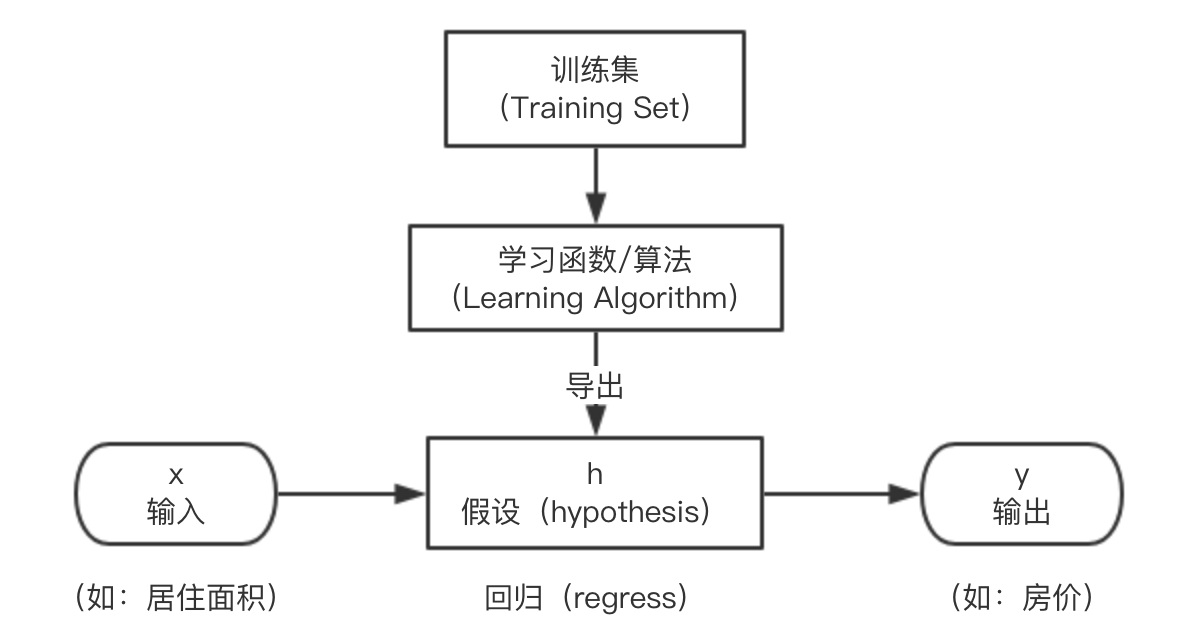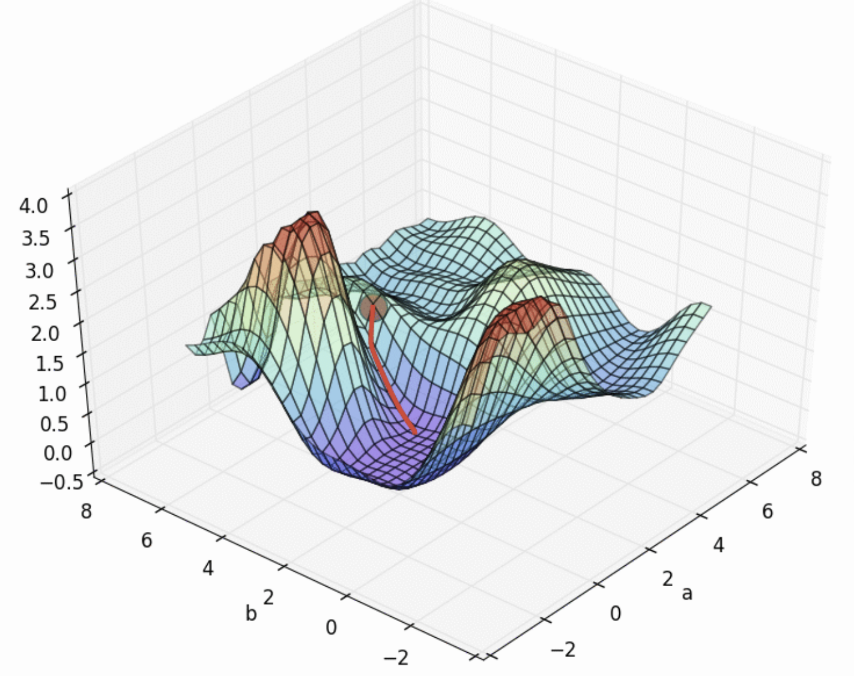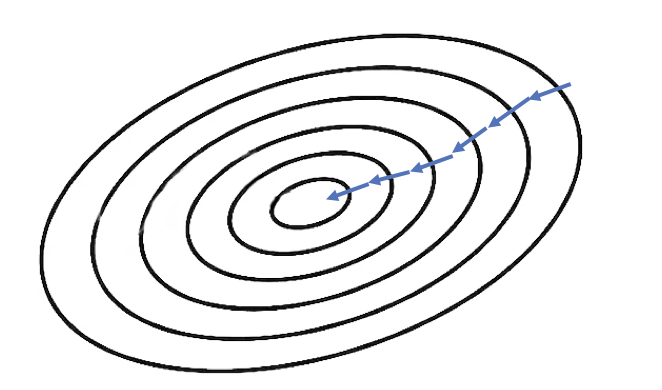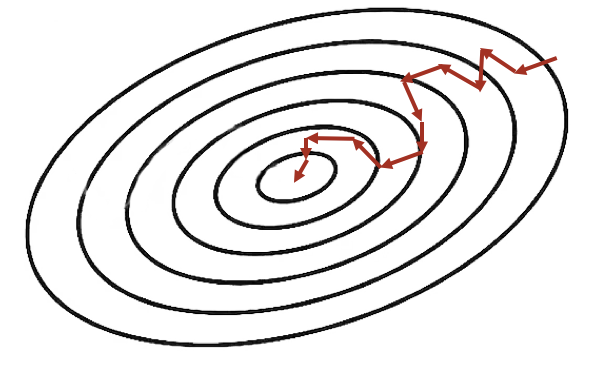## 监督学习

### 符号定义：

$m$ 训练集包含的数据个数
$x$ 输入变量/特征（feature）
$y$ 输出变量/目标（target）
$(x, y)$ 一个训连样本
$(x^{(i)}, y^{(i)})$ 第 i 个训练样本

### 监督学习的主要流程：### 线性回归

• 输入（特征）：$x_1, x_2, …$（比如大小、卧室数量等等）
• 输出（目标）：$y$（房价）
• 假设：$h(x)=h_{\theta}(x)=\theta_0+\theta_1x_1+\theta_2x_2+…+\theta_nx_n$，用于预测房价，其中$\theta_i$是参数，$n$是特征数量

为了方便，可以将假设写成：$h(x)=\sum_{i=0}^n\theta_ix_i=\theta^Tx​$

## 梯度下降法

### 搜索算法（梯度下降）